# Convert given Binary Search Tree to a Greater Sum Tree

In this article, we are going to see how to convert a given Binary search tree to a greater sum tree?
Submitted by Radib Kar, on October 11, 2020

In this article, we are going to see how we can convert a binary search tree into a greater sum tree? Before discussing the solution, let’s understand what does mean by a greater sum tree. A greater sum tree means the root will have value same as the sum of the nodes having value more than the root. Let’s discuss that with an example.

Below is the binary tree to be considered: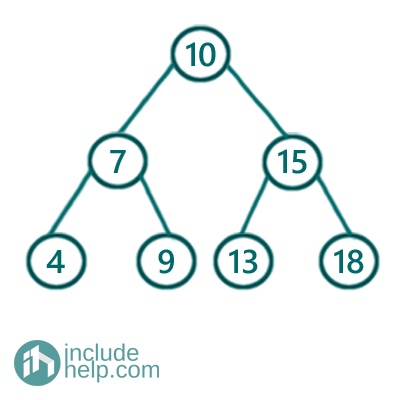For example, the rightmost node 18 doesn't have any value greater than it, so that will be replaced by 0. On the other has node 13 has nodes 15 & 18 greater than that, so that will be replaced by 33. If we continue this way the resulting subtree will be: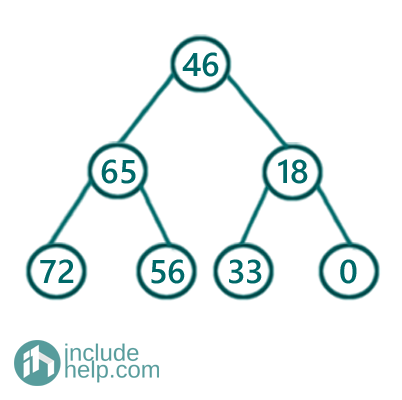Solution:

Of course, there is a naïve way, where for each node we can find which nodes are greater than this and can replace the node value with the sum the greater nodes. That will take O(n^2) time complexity and similar for the binary tree as well. But we can have a better one pass solution using the properties of the binary search tree. The thing is that to visit the tree nodes in reverse sorted order(descending) we need reverse inorder traversal. And we can use that reverse inorder traversal to solve. The algorithm is like below:

1. Have a variable sum which will store sum while the reverse inorder traversal. Initiate sum to 0.
2. Do reverse inorder traversal like below:
1. ```// Base case
if root is NULL
return;
```
2. Recur for right subtree first as it's a reverse inorder traversal.
3. store the current sum to variable previous sum and update sum as sum+ root value.
Change the root value as previous sum which is the sum of the nodes already traversed and those are surely greater than the node since they fall in right subtree only.

4. Recur for left subtree now.

Below is the implementation of the above algorithm:

```#include <bits/stdc++.h>
using namespace std;

// tree node is defined
class TreeNode {
public:
int val;
TreeNode* left;
TreeNode* right;
TreeNode(int data)
{
val = data;
left = NULL;
right = NULL;
}
};

//inorder traversal for tree
void inorder(TreeNode* root)
{

if (!root)
return;
inorder(root->left);
cout << root->val << " ";
inorder(root->right);
}

// Recursive function to transform a BST to sum tree.
// do a reverse inorder traversal for the BST
void transformToGreaterSumTreeRecur(TreeNode* root, int& sum)
{
// Base case
if (!root)
return;

// Recur for right subtree first as
//it's reverse inorder traversal
transformToGreaterSumTreeRecur(root->right, sum);

// store the previous sum and update sum
int prevSum = sum;
sum = sum + root->val;

// Store old sum in the current node which is sum
// of the right subtree nodes(nodes greater than the root)
root->val = prevSum;

// Recur for left subtree now
transformToGreaterSumTreeRecur(root->left, sum);
}

void transformToGreaterSumTree(TreeNode* root)
{
//initially sum is 0
int sum = 0;
transformToGreaterSumTreeRecur(root, sum);
}

int main()
{
//Tree built as per example
TreeNode* root = new TreeNode(10);

root->left = new TreeNode(7);
root->right = new TreeNode(15);
root->left->left = new TreeNode(4);
root->left->right = new TreeNode(9);
root->right->left = new TreeNode(13);
root->right->right = new TreeNode(18);

cout << "Inorder Traversal of the given tree\n";
inorder(root);
cout << "\nconverting to greater Sum tree\n";
transformToGreaterSumTree(root);
cout << "Conversion completed\n";
cout << "Inorder Traversal of transformed greater sum tree:\n";
inorder(root);

return 0;
}
```

Output:

```Inorder Traversal of the given tree
4 7 9 10 13 15 18
Converting to greater Sum tree
Conversion completed
Inorder Traversal of transformed greater sum tree:
72 65 56 46 33 18 0
```

Dry run:

Below is the step by step dry run of the above implementation. Since it's a reverse inorder traversal, it will first reach the rightmost node.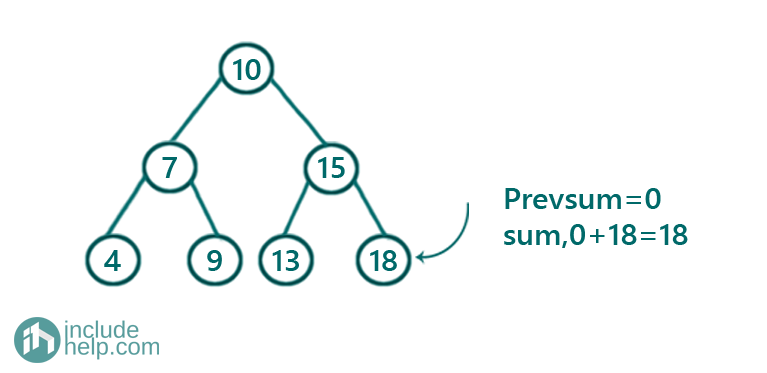Initially, sum is 0 so that will be transferred to the rightmost node via prevsum and the sum updated as sum+root->val=0+18=18.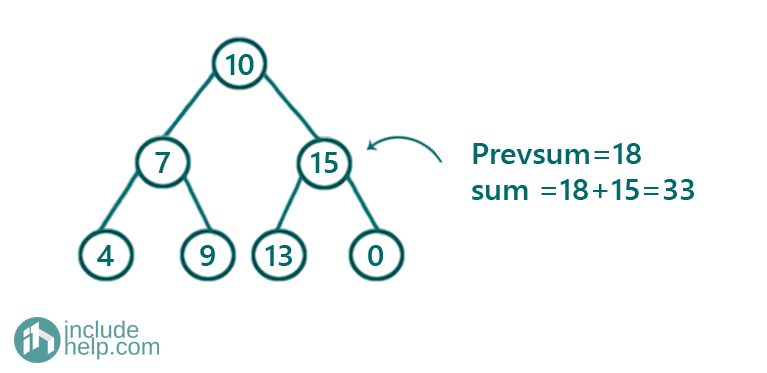Now, sum is 18 so that will be transferred to the current node via prevsum and the sum updated as sum+root->val=18+15=33.

You can check that 18 is the only greater node than 15.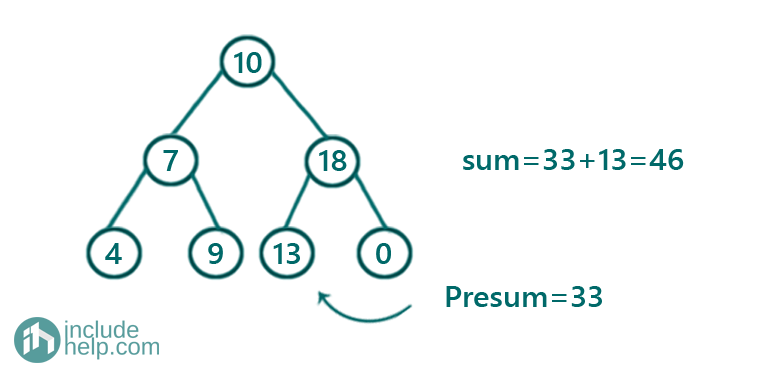Now, sum is 33 so that will be transferred to the current node via prevsum and the sum updated as sum+root->val=33+13=46.

You can check that 18 & 15(total 33) are the only greater node than 13.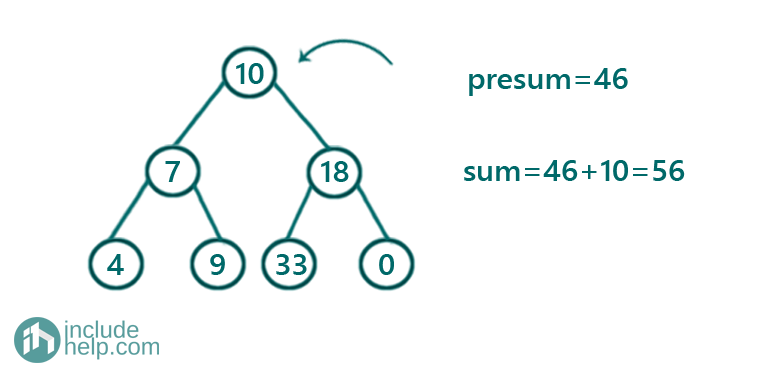Now, sum is 46 so that will be transferred to the current node via prevsum and the sum updated as sum+root->val=46+10=56.

You can check that 18, 15, 13(total 46) are the greater nodes than 10.

Similarly,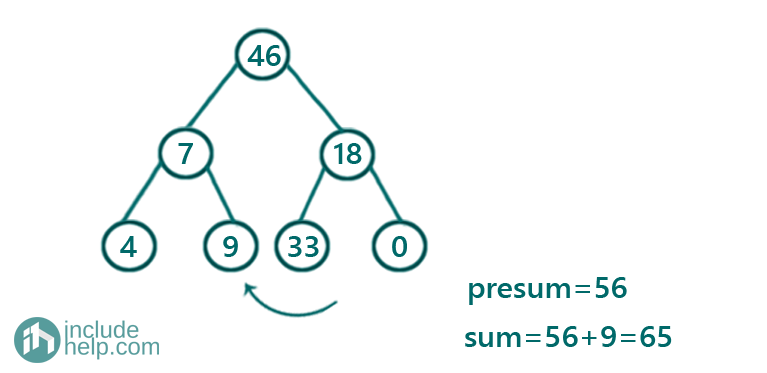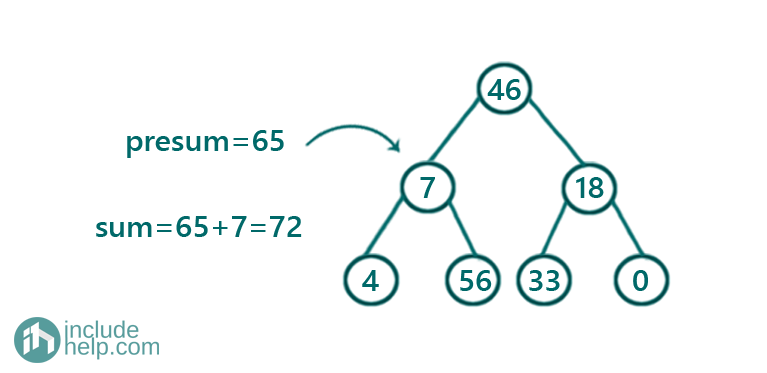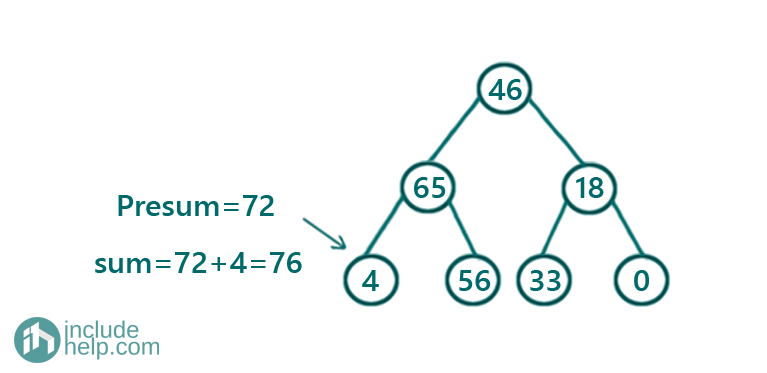Finally, the greater sum tree is:Languages: » C » C++ » C++ STL » Java » Data Structure » C#.Net » Android » Kotlin » SQL
Web Technologies: » PHP » Python » JavaScript » CSS » Ajax » Node.js » Web programming/HTML
Solved programs: » C » C++ » DS » Java » C#
Aptitude que. & ans.: » C » C++ » Java » DBMS
Interview que. & ans.: » C » Embedded C » Java » SEO » HR
CS Subjects: » CS Basics » O.S. » Networks » DBMS » Embedded Systems » Cloud Computing
» Machine learning » CS Organizations » Linux » DOS
More: » Articles » Puzzles » News/Updates